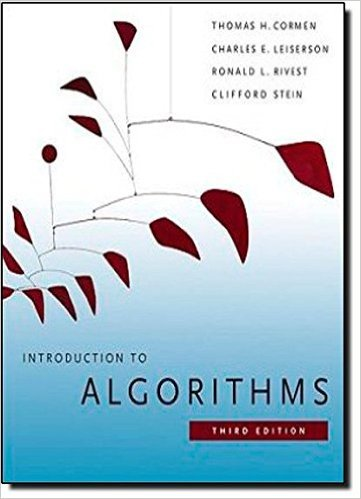×
Get Full Access to Introduction To Algorithms - 3 Edition - Chapter 22 - Problem 22-2
Get Full Access to Introduction To Algorithms - 3 Edition - Chapter 22 - Problem 22-2

×

# Articulation points, bridges, and biconnected componentsISBN: 9780262033848 130

## Solution for problem 22-2 Chapter 22

Introduction to Algorithms | 3rd Edition

• Textbook Solutions
• 2901 Step-by-step solutions solved by professors and subject experts
• Get 24/7 help from StudySoup virtual teaching assistantsIntroduction to Algorithms | 3rd Edition

4 5 1 414 Reviews
13
3
Problem 22-2

Articulation points, bridges, and biconnected components Let G D .V; E/ be a connected, undirected graph. An articulation point of G is a vertex whose removal disconnects G. A bridge of G is an edge whose removal disconnects G. A biconnected component of G is a maximal set of edges such that any two edges in the set lie on a common simple cycle. Figure 22.10 illustrates 1 2 3 4 5 6 Figure 22.10 The articulation points, bridges, and biconnected components of a connected, undirected graph for use in 22-2. The articulation points are the heavily shaded vertices, the bridges are the heavily shaded edges, and the biconnected components are the edges in the shaded regions, with a bcc numbering shown. these definitions. We can determine articulation points, bridges, and biconnected components using depth-first search. Let G D .V; E/ be a depth-first tree of G. a. Prove that the root of G is an articulation point of G if and only if it has at least two children in G . b. Let be a nonroot vertex of G. Prove that is an articulation point of G if and only if has a child s such that there is no back edge from s or any descendant of s to a proper ancestor of . c. Let :low D min ( :d ; w:d W .u; w/ is a back edge for some descendant u of : Show how to compute :low for all vertices 2 V in O.E/ time. d. Show how to compute all articulation points in O.E/ time. e. Prove that an edge of G is a bridge if and only if it does not lie on any simple cycle of G. f. Show how to compute all the bridges of G in O.E/ time. g. Prove that the biconnected components of G partition the nonbridge edges of G. h. Give an O.E/-time algorithm to label each edge e of G with a positive integer e:bcc such that e:bcc D e0 :bcc if and only if e and e0 are in the same biconnected component.

Step-by-Step Solution:
Step 1 of 3

MTH 132 - Lecture 20 - Mean THM Mean Value Theorem 1. f(x) is continuous on the interval [a,b] 2. f(x) is differentiable on the interval [a,b] ● When Ǝ c ε(a,b) such that f(c) = [ f(b) - f(a) ] / [b - a] ● Slope = [ f(b) - f(a) ] / [b - a] Rolle's Theorem ● if f(x) is continuous on the closed interval [a, b] and differentiable on the open interval (a, b) such that f(a) = f(b), then f′(x) = 0 for some x with a ≤ x ≤ b. Proof: ● Idea: MVT = changed Rolle's THM. ● Let g(x) = f(x) - [f(a) + [ f(b) - f(a) ] / [b - a] * (x-a) ] ● The line goes through [a,f(a)] and [b,f(b)] ● g(a) = f(a) - f(a) = 0 ● g(b) = f(b) - f(a) - [f(b)- f(a)] = 0 ● We can use Rolle's THM Ǝ c ε(a,b) such that g’(c) = 0. Example: ● 5 - 6x^2 m[-6

Step 2 of 3

Step 3 of 3

##### ISBN: 9780262033848

Unlock Textbook Solution